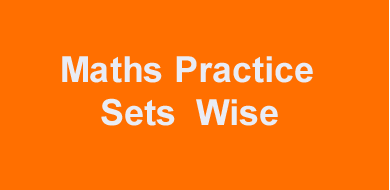# Math simplification or calculation practice 2 related questions for all exam#### Math simplification questions for bank PO clerk exam for practice set IBPS PO, SBI, RRB Quiz and special list officer exam Quantitative Aptitude test for SSC CGL Clerk LDC .We are daily upload the math related question Quantitative Aptitude Quiz. Simplification or calculation topic is very important for all bank exam.

Number of students preparation for Bank related SSC and other compitetive related exam such as IBPS SBI RRB PO clerk SSC CGL clerk.Some topic is very importent in all exam such as  Simplification and calculation topic, Number Series topic, Permutation & Combination topic, Quadratic Equation topic,  Data Interpretation topic, Data Analysis topic

## Simplification questions practice set for competitive exam

Questions  (0.76 × 0.76 × 0.76 − 0.008)/(0.76 × 0.76 + 0.76 × 0.2 + 0.04)

Daily Visit GK on myshort.in

A. 0.52

B. 0.54

C. 0.56

D. 0.57

Ans . 0.56

Questions   0.8 × 42 − 36 × 0.7 = ?

A. 8.6

B. 8.5

C. 8.4

D. 8.2

Ans 8.4

Questions  2/7 of 105 = ? × 90

A. 1/3

B. 1/8

C. 1/2

D. 1/7

Ans 1/3

Questions  (1024 − 263 − 233) ÷ (986 − 764 − 156) = ?

A. 8

B. 7

C. 6

D. 4

Ans 8

Questions  √(625) ÷ 5 − ? = 6265

A. 1253

B. 1254

C. 1257

D. 1258

Ans 1253

Questions  832/24 × 84/26÷252/72=?

A. 31

B. 34

C. 37

D. 32

C.) 32

Questions  (96×√49)/√196=?×√36

A. 8

B. 2

C. 4

D. 6

Ans 8

Questions  326-441+1130=?-141

A. 871

B. 872

C. 874

D. 877

Ans 874

Questions  0.2 + 1.3 – 0.4 × 1.5

A. 0.7

B. 0.1

C. 0.2

D. 0.0

Ans 0.9

Questions  0.3 × 0.4 – 2.4 ÷ 6 + 1.2 × 4

A. 4.52

B. 4.57

C. 4.51

D. 4.50

Ans 4.52

Questions  0.72 ÷ 1.2 + 3.5 × 4.2 – 1.6

A. 13.2

B. 13.4

C. 13.7

D. 13.1

Ans 13.7

Questions  9.2 + 3.5 – 4.9 – 3.5 ÷ 0.7 × 1.2

A. 1.8

B. 1.7

C. 1.6

D. 1.2

Ans 1.8

Questions  5.01 × 1.2 – 2.4 ÷ 2

A. 4.812

B. 5.812

C. 6.812

D. 2.812

Ans 4.812

Questions 8195 ÷ 745 + ? × 12 = 7847

A. 652

B. 651

C. 656

D. 653

Ans 653

Questions (14896 ÷ 19) ÷ 16 = ?

A. 49

B. 44

C. 45

D. 47

Ans 49

Questions 8195 ÷ 745 + ? × 12 = 7847

A. 650

B. 653

C. 652

D. 651

Ans 653

Questions 12.25 × 7.2 + 84.33 = ?

A. 182.21

B. 182.41

C. 182.11

D. 182.51

Ans 182.51

Questions 9643 – 7750 + ? = 4990

A. 3091

B. 3092

C. 3094

D. 3097

Ans 3097

Questions 98643 – 21748 = 51212 + ?

A. 35683

B. 45683

C. 55683

D. 25683

Ans 25683

Questions 6389 – 1212 – 2828 = ?

A. 2342

B. 2349

C. 2341

D. 2346

Ans 2349

Questions 100 × 10 – 100 + 2000 ÷ 100 = ?

A. 921

B. 924

C. 922

D. 920

Ans 920

Questions 5004 ÷ 139 – 6 = ?

Buy online Rajasthan gk book Railway JE CBT REET PAtwari Book SSC CGL Clerk GD Book Buy online Rajasthan gk book Railway JE CBT REET PAtwari Book SSC CGL Clerk GD Book